Grade - examples - page 158

1. RavensOn two trees sitting 17 ravens. If 3 ravens flew from first to second tree and 5 ravens took off from second tree then the first tree has 2 times more ravens than second tree. How many ravens was originally on every tree?
2. Spain vs USASpain lost to the US by 4 goals. In the match total fell 10 goals. How many goals gave the Spain and how the United States?
3. Kerosine and petrol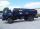if 4 litres of petrol containing 15% kerosine are added to another 7 litres of petrol containing 10% kerosine, what percentage of the petrol is kerosine?
4. Fifth memberDetermine the fifth member of the arithmetic progression, if the sum of the second and fifth members equal to 73, and difference d = 7.
5. CandiesThere are red, blue and green candies in bad. Red to green is in 6:11 ratio and blue to red in a 7: 5 ratio. In what proportion are blue to green candies?
6. Waste paper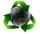Our school attend 1,300 pupils. Each pupil collected plastic bottles or paper. Plastic bottles have collected 930 pupils and 600 pupils old paper. How many students have collected together plastic bottles and waste paper?
7. The bunsKate, Zofia and Peter Liked buns. Even today, their grandmother prepare their favorite meal. Katka eats 4 bunches, Žofia 3 and Petra eats 5 buns. Their grandmother said to them, "My inmate will you know how many buns I have been make today, if those you ea
8. Rectangle 35Find the area of a rectangle when the diagonal is equal to 30 cms and the width is double the length.
9. Tulips and daffodils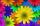Farm cultivated tulips and 211 units on 50 units more daffodils. How many spring flowers grown together?
10. The rectangleDetermine the area of the rectangle where the length and width are in the ratio 5:2 and its length is 7.5 cm longer than its width. Determine also its length and its width.
11. Unknown number x5% from an unknown number equals the number 26. What is the unknown number?
12. Cross sectionThe cross-section ABCD of a swimming pool is a trapezium. Its width AB=14 meters, depth at the shallow end is 1.5 meters and at the deep end is 8 meter. Find the area of the cross-section.
13. ApplesApples I picked 600 kg this year. Autumn's apples was 4 times more than the summer. How many kg's I picked the summer and how much winter apples?
14. Pond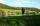A pond with two open floodgates will emptied in 20 days. A larger floodgate will emptied whole pond in 30 days. For how long would he be emptied with a smaller floodgate.
15. Guess a fractionTom was asked to guess a fraction. The sum of 1/2 the numerator and 1/3 of its denominator is 30. If Tom subtracts 36 from its denominator, the fraction becomes 1/3. What is the fraction that Tom was asked to guess? (Leave your answer in simplest form)
16. Ratio - proportion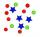Reduce the number 16 in proportion 3:2 5:4 11:8On a straight stretch of road is marked 12 percent drop. What angle makes the direction of the road with the horizontal plane?x walnuts were in the mission. Dano took 1/4 of nuts Michael took 1/8 from the rest and John took 34 nuts. It stayed here 29 nuts. Determine the original number of nuts.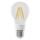One percent of all the lights in the city are LED, the remaining 99% are conventional. Other types are not there. John counted them honestly but he had counted only conventional. After a good dinner he registered numbers and he have notice that from all tAre these numbers 2i, 4i, 2i + 1, 8i, 2i + 3, 4 + 7i, 8i, 8i + 4, 5i, 6i, 3i complex?